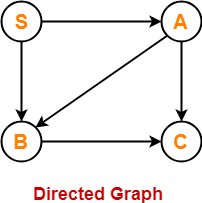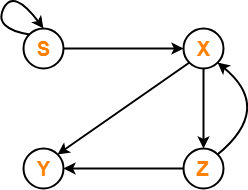## Context Free Language-

Before you go through this article, make sure that you have gone through the previous article on Context Free Language.

We have discussed-

• Context free language is generated using a context free grammar.
• Each context free language is accepted by a Pushdown automaton.

## Algorithm To Decide Whether CFL Is Finite Or Not-

For a given CFG, there exists an algorithm to decide whether its language is finite or not.

### Step-01:

Reduce the given grammar completely by-

• Eliminating ∈ productions
• Eliminating unit productions
• Eliminating useless productions

Also Watch- How To Reduce Grammar?

### Step-02:

• Draw a directed graph whose nodes are variables of the given grammar.
• There exists an edge from node A to node B if there exists a production of the form A → αBβ.

Now, following 2 cases are possible-

### Case-01:

• Directed graph contains a cycle.
• In this case, language of the given grammar is infinite.

### Case-02:

• Directed graph does not contain any cycle.
• In this case, language of the given grammar is finite.

Also Read- Algorithm To Decide Whether CFL Is Empty

## Problem-01:

Check whether language of the following grammar is finite or not-

S → AB / a

A → BC / b

B → CC / c

## Solution-

### Step-01:

The given grammar is already completely reduced.

### Step-02:

We will draw a directed graph whose nodes will be S , A , B , C.

Now,

• Due to the production S → AB, directed graph will have edges S → A and S → B.
• Due to the production A → BC, directed graph will have edges A → B and A → C.
• Due to the production B → CC, directed graph will have edge B → C.

The required directed graph is-Clearly,

• The directed graph does not contain any cycle.
• Therefore, language of the given grammar is finite.

## Problem-02:

Check whether language of the following grammar is finite or not-

S → XS / b

X → YZ

Y → ab

Z → XY

## Solution-

### Step-01:

The given grammar is already completely reduced.

### Step-02:

We will draw a directed graph whose nodes will be S , X , Y , Z.

Now,

• Due to the production S → XS / b, directed graph will have edges S → X and S → S.
• Due to the production X → YZ, directed graph will have edges X → Y and X → Z.
• Due to the production Z → XY, directed graph will have edges Z → X and Z → Y.

The required directed graph is-Clearly,

• The directed graph contain cycles.
• Therefore, language of the given grammar is infinite.

Next Article- CYK Algorithm

Get more notes and other study material of Theory of Automata and Computation.

Watch video lectures by visiting our YouTube channel LearnVidFun.